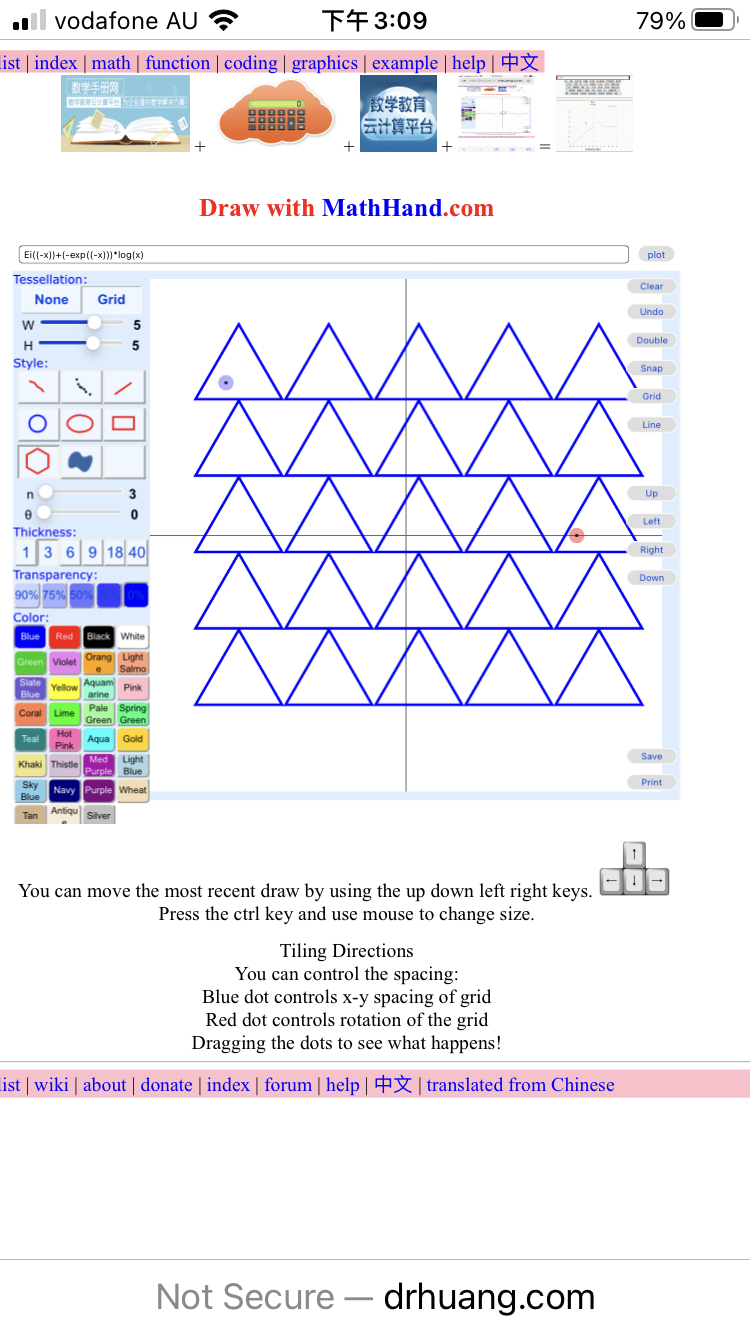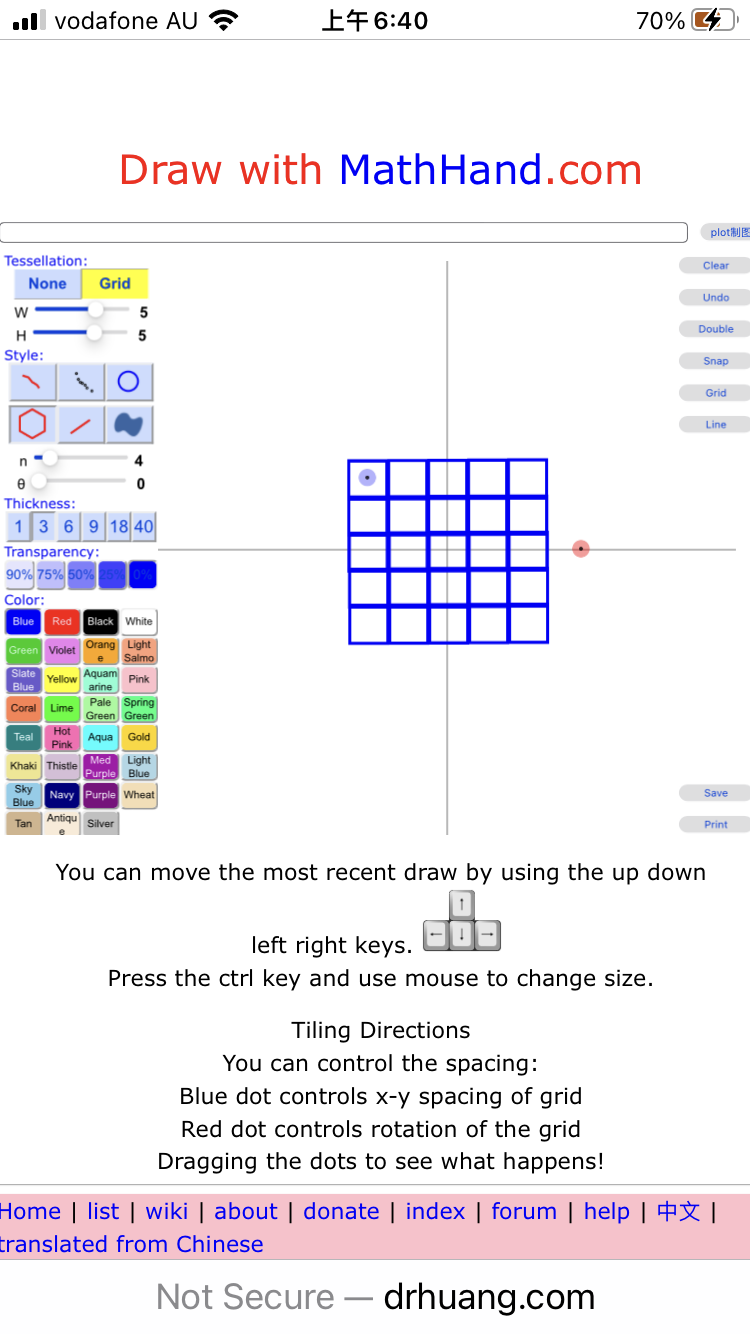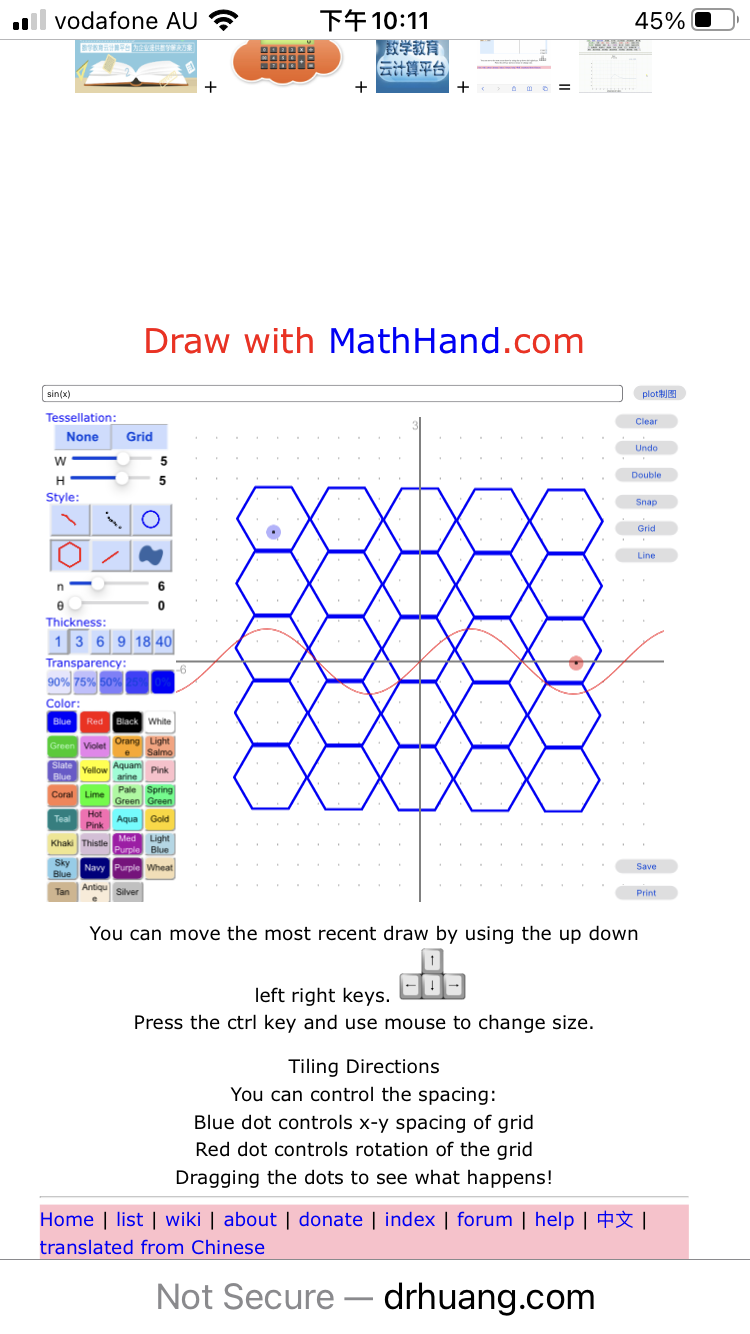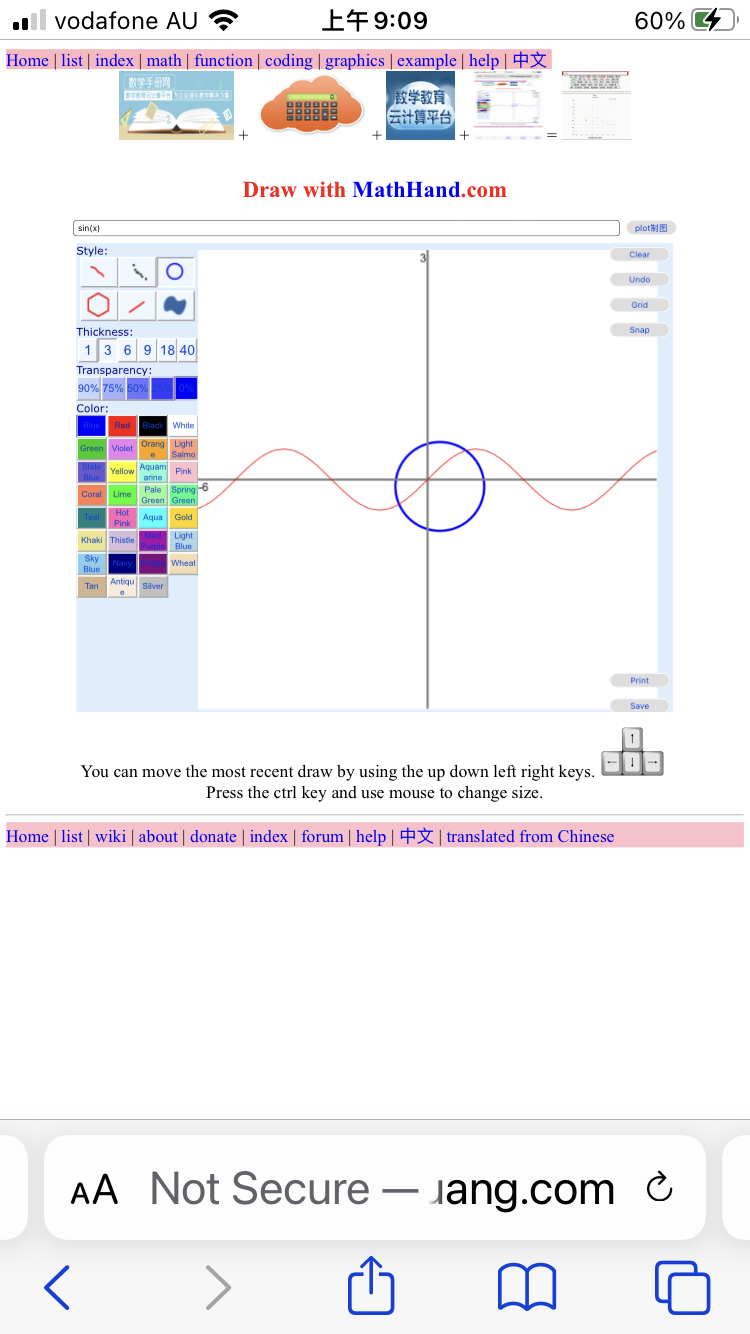﻿﻿ Fractional Calculus Computer Algebra System, Electrochemistry Software, Massage+++=﻿

# Drawing 画画

## Drawing

Drawing with the online software draw
1.2.3.4. A function sin(x) curve which is overlap plot.5. A function sin(x)curve which is overlap plot.## Reference 参阅

• example 例题
• function graph 制图
• graphics 制图法
• animation 动画 ﻿